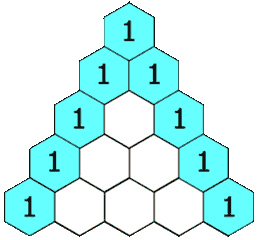# GeetCode Hub

Given an integer `numRows`, return the first numRows of Pascal's triangle.

In Pascal's triangle, each number is the sum of the two numbers directly above it as shown:Example 1:

```Input: numRows = 5
Output: [,[1,1],[1,2,1],[1,3,3,1],[1,4,6,4,1]]
```

Example 2:

```Input: numRows = 1
Output: []
```

Constraints:

• `1 <= numRows <= 30`

class Solution { public List<List<Integer>> generate(int numRows) { } }